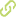# Test of Understanding Graphs in Calculus: Test of students' interpretation of calculus graphs•
• Overview
•
• Identity
•
•
• View All
•

### Abstract

• © Authors.Studies show that students, within the context of mathematics and science, have difficulties understanding the concepts of the derivative as the slope and the concept of the antiderivative as the area under the curve. In this article, we present the Test of Understanding Graphs in Calculus (TUG-C), an assessment tool that will help to evaluate students' understanding of these two concepts by a graphical representation. Data from 144 students of introductory courses of physics and mathematics at a university was collected and analyzed. To evaluate the reliability and discriminatory power of this test, we used statistical techniques for individual items and the test as a whole, and proved that the test's results are satisfactory within the standard requirements. We present the design process in this paper and the test in the appendix. We discuss the findings of our research, students' understanding of the relations between these two concepts, using this new multiple-choice test. Finally, we outline specific recommendations. The analysis and recommendations can be used by mathematics or science education researchers, and by teachers that teach these concepts.

### Publication date

• January 1, 2017